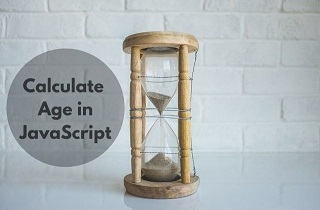# How to Calculate Age from DOB in JavaScript

JavaScript allows you to do many fun things with dates and strings. Sometimes you may need to calculate age from date of birth to display the information on your websites. In this article, we will learn how to calculate age from DOB in JavaScript.

## How to Calculate Age from DOB in JavaScript

Let us say you have the following date string in format YYYYMMDD.

`var d = '19850501'`

First of all, we will obtain date from above date string.

`var b = Date(d);`

Next, we will obtain today’s date.

`var t = Date();`

Next, we calculate age by first calculating difference in years between today’s date and given birthdate. We use getFullYear() function for this purpose. It returns the full 4 digit year as a number.

`var y = t.getFullYear() - b.getFullYear();`

Next, we calculate month difference between two months. We use getMonth() function for this purpose. It gives month number.

`var m = t.getMonth() - b.getMonth();`

If the birth date is in future then we need to subtract one from difference of years.

```if (m < 0 || (m === 0 && t.getDate() < b.getDate())) {
y--;
}```

Putting it all together,

```function getAge(d) {
var t = new Date();
var b = new Date(d);
var y = t.getFullYear() - b.getFullYear();
var m = t.getMonth() - b.getMonth();
if (m < 0 || (m === 0 && t.getDate() < b.getDate())) {
y--;
}
return y;
}```

Alternatively, you can also first get difference of date from present date time.

`var age_diff = Date.now() - b.getTime();`

Next, we calculate difference from epoch.

`var diff_date = new Date(age_diff);`

Finally, we calculate the difference from epoch in years.

`Math.abs(diff_date.getUTCFullYear() - 1970);`

Putting it all together,

```function getAge(d) {
var b = new Date(d);
var age_diff = Date.now() - b.getTime();
var diff_date = new Date(age_diff);
return Math.abs(diff_date.getUTCFullYear() - 1970);
}```

In this article, we have learnt how to calculate age from DOB in JavaScript. You can use this to calculate age from date strings on your website/application.